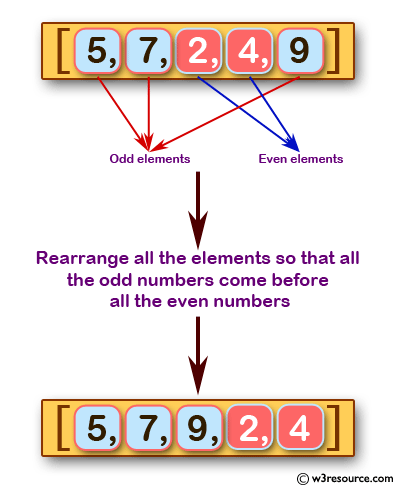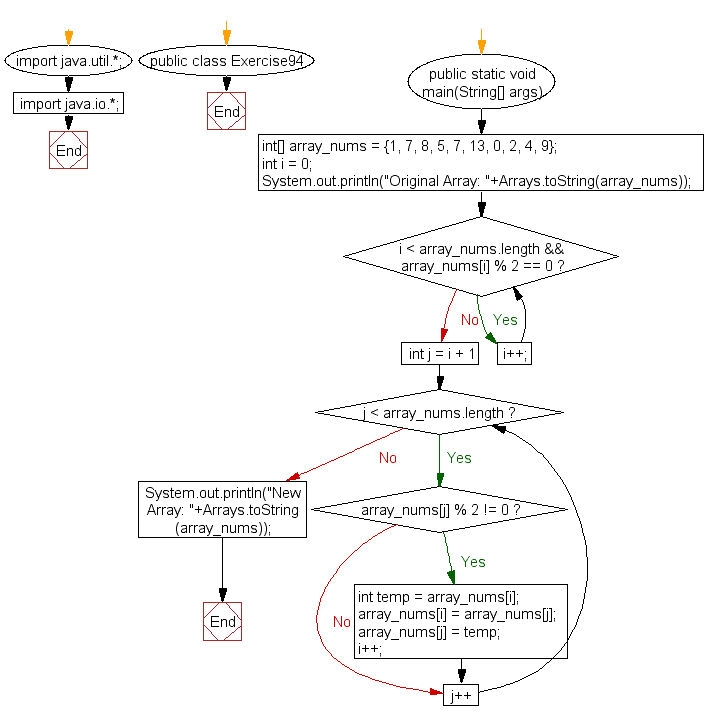﻿ Java exercises: Rearrange all the elements of an given array of integers so that all the odd numbers come before all the even numbers - w3resource# Java Exercises: Rearrange all the elements of an given array of integers so that all the odd numbers come before all the even numbers

## Java Basic: Exercise-94 with Solution

Write a Java program to rearrange all the elements of an given array of integers so that all the odd numbers come before all the even numbers.

Pictorial Presentation:Sample Solution:

Java Code:

``````import java.util.*;
import java.io.*;
public class Exercise94 {
public static void main(String[] args)
{
int[] array_nums = {1, 7, 8, 5, 7, 13, 0, 2, 4, 9};

int i = 0;
System.out.println("Original Array: "+Arrays.toString(array_nums));
while(i < array_nums.length && array_nums[i] % 2 == 0)
i++;

for(int j = i + 1; j < array_nums.length; j++) {
if(array_nums[j] % 2 != 0) {
int temp = array_nums[i];
array_nums[i] = array_nums[j];
array_nums[j] = temp;
i++;
}
}

System.out.println("New Array: "+Arrays.toString(array_nums));
}
}
```
```

Sample Output:

```Original Array: [1, 7, 8, 5, 7, 13, 0, 2, 4, 9]
New Array: [7, 5, 7, 13, 9, 1, 0, 2, 4, 8]
```

Flowchart:Java Code Editor:

What is the difficulty level of this exercise?

﻿

## Java: Tips of the Day

initial:

Returns all the elements of an array except the last one. Use Arrays.copyOfRange() to return all except the last one

```public static <T> T[] initial(T[] elements) {
return Arrays.copyOfRange(elements, 0, elements.length - 1);
}
```

Ref: https://bit.ly/3zEKw9u